# Lambda Expressions in C++

## Lambda Expressions in C++ with Examples:

In this article, I am going to discuss Lambda Expressions in C++ with Examples. Please read our previous article where we discussed Final Keyword in C++ with Examples. The Lambda Expressions was Introduced in C++ 11.

##### Lambda Expressions in C++

I Lambda Expressions is useful for defining unnamed functions in C++. So, we can define the function wherever we like. They are more likely inline functions but they will have the features of the function. Let us see the syntax for a lambda expression or unnamed function in C++.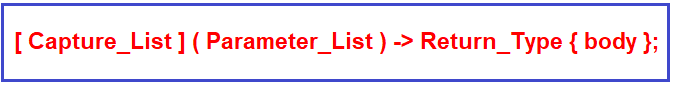This is the syntax for writing an unnamed function using Lambda Expression in C++. Let us see how to write these functions and how to call these functions in C++ with Examples. And we will see what is the use of lambda expressions and how powerful they are. Please have a look at the following example code.

```#include <iostream>
using namespace std;
int main(){
[](){cout << "Hello"<<endl;}();
[](int x, int y) {cout << "Sum: " << x + y <<endl;}(10, 5);
int x = [](int x, int y) {return x+y;} (10, 5);
cout<<x;
}
```
###### Output: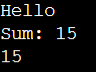Inside the main function, we have written some statements. Let us understand them.

[](){cout << “Hello”<<endl;}(); In the 1st statement, there is no capture list, no parameter list. It is just the body that will display Hello in the console. So, for calling this function we can directly put the parenthesis ‘()’ after the curly braces. So, we have defined the function as well as called the function in the same statement.

[](int x, int y) {cout << “Sum: ” << x + y <<endl;}(10, 5); In the 2nd statement, we have defined another function and in that function, there is no capture list but the function is taking two integer parameters x and y and in the body part, we have written a print statement that will print the sum of these two parameters. After the closing curly brace, we have given parameters 10 and 5 inside the parenthesis. So, by writing parenthesis after curly braces, we can call the function. Here 10 will be passed to x and 5 will be passed to y then it will display the sum of these numbers in the console.

int x = [](int x, int y) {return x+y;} (10, 5); In the 3rd statement, we have written a function that will return some value. The function is taking 2 parameters and it will return the sum of the parameters. We have stored the result of this function inside the x variable which is of int type.

##### Another way of Calling Unnamed Function in C++:

Now, instead of calling the function at the defining time, we can also assign that function to some variable. For example,

auto F = [](){cout << “Hello”;};

Here the unnamed function is assigned to the F. Now, the name of the function is F. Though the function is unnamed but F is a reference to that function. We can call this function by using the name F. For example,

F();

This statement will call the function. So, we have assigned the function to some reference i.e. F which is an auto reference or pointer to a function. So, by using pointer F we have called the function. The complete example code is given below.

```#include <iostream>
using namespace std;
int main(){
auto F =  [](){cout << "Hello";};
F();
}
```

So, we can directly call the function by passing parameters, or else we can assign that function to some reference, and then by using that reference we can also call that function. Now let us see how we can write the return type.

##### Writing Return Type using Lambda Expression in C++:

By default, the C++ compiler will identify the return type, so we don’t have to mention it or if you want then you can mention it. For example,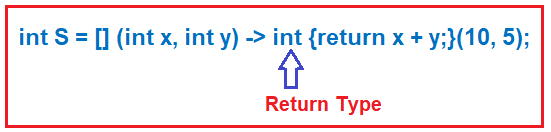Here, we have defined an unnamed function that is taking 2 integer type parameters i.e. x and y, and then we have given the return type i.e. int. At the defining time, we have also called this function by giving some parameters 10 and 5 and storing the result in the S variable. Here, we have explicitly mentioned the return type of this unnamed method as int. The complete example code is given below.

```#include <iostream>
using namespace std;
int main(){
int S = [] (int x, int y) -> int{return x + y;}(10, 5);
cout<<S;
}
```

Output: 15

##### Can we access local variables of a function inside the unnamed function in C++?

Yes, we can access the local variables of a function inside the unnamed function in C++ using the captured list. Let us see one example to understand this concept. Please have a look at the following example.

```#include <iostream>
using namespace std;
int main(){
int x = 3;
int y = 6;
[] () {cout << x << " " << y};
}
```

Inside the main function, we have 2 variables x and y with some values. So, can we access these variables in the unnamed function? Let us see. We have written a lambda expression or unnamed function in which we are printing the value of x and y. So, we are expecting that this function should display the value of x and y. But it will not display rather it will give us the following error.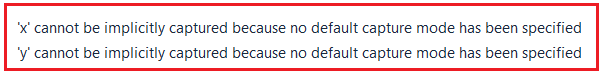What it means is that we cannot implicitly capture the x and y variables. That means We have to capture these variables in the capture list of the Lambda Expression. Because directly they are not accessible. So let us write the function with the capture list as follows:

[x, y] () {cout << x << ” ” << y;};

So, we have written the same function but here it is taking x and y as capture list. Now x and y are accessible. See here we defined an unnamed function inside the main function so it is more like a nested function. The complete example code is given below.

```#include <iostream>
using namespace std;
int main(){
int x = 3;
int y = 6;
[x, y] () {cout << x << " " << y;}();
}
```

Output: 3 6

[x, y] () {cout << ++x << ” ” << ++y;}; Can we write like this? No. We cannot modify these captured variables. For modifying, we have to use a reference for capturing variables as follows:

[&x, &y] () {cout << ++x << ” ” << ++y;};

Now we can modify the x and y values. By writing reference, we can modify the local variables inside the lambda expression. The complete example code is given below.

```#include <iostream>
using namespace std;
int main(){
int x = 3;
int y = 6;
[&x, &y] () {cout << ++x << " " << ++y;}();
}
```

Output: 4 7

Now if we want to access all the things in this scope then just write a reference inside the capture list as follows:

[&] () {cout << ++x << ” ” << ++y;};

Now we can modify all the values. The complete example code is given below.

```#include <iostream>
using namespace std;
int main(){
int x = 3;
int y = 6;
[&] () {cout << ++x << " " << ++y;}();
}
```

Output: 4 7

##### Example to Understand Lambda Expression in C++:
```#include <iostream>
using namespace std;

template<typename t>
void fun(t r){
r();
}

int main(){
cout << "Function 1:" << endl;
[](){cout << "Hello";}();

cout << endl << "Function 2:" << endl;
int z = [](int x, int y) {return x+y;} (30, 32);
cout << "Sum: " << z;

cout << endl << "Function 3:" << endl;
int x = 5;
int y = 6;

auto F = [&x, &y](){cout << ++x << " " << ++y << endl;};
cout << "x & y : ";
F();

cout << endl << "Function 4:" << endl;
auto S = [x, y](){cout << "x * y: " << x*y << endl;};
fun(S);
}
```
###### Output: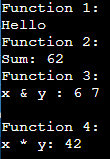In the next article, I am going to discuss Smart Pointers in C++ with Examples. Here, in this article, I try to explain Lambda Expression in C++ with Examples and I hope you enjoy this article. I would like to have your feedback. Please post your feedback, question, or comments about Lambda Expression in C++ with the Examples article.